# Kolmogorov-Smirnov test

(diff) ← Older revision | Latest revision (diff) | Newer revision → (diff)

A non-parametric test used for testing a hypothesis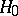, according to which independent random variableshave a given continuous distribution function, against the one-sided alternative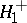: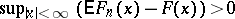, where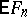is the mathematical expectation of the empirical distribution function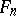. The Kolmogorov–Smirnov test is constructed from the statistic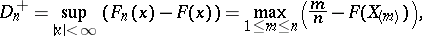whereis the variational series (or set of order statistics) obtained from the sample. Thus, the Kolmogorov–Smirnov test is a variant of the Kolmogorov test for testing the hypothesisagainst a one-sided alternative. By studying the distribution of the statistic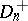, N.V. Smirnov  showed that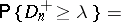(1)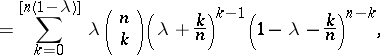where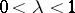and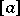is the integer part of the number. Smirnov obtained in addition to the exact distribution (1) of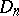its limit distribution, namely: Ifand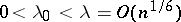, thenwhereis any positive number. By means of the technique of asymptotic Pearson transformation it has been proved  that ifand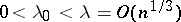, then(2)

According to the Kolmogorov–Smirnov test, the hypothesismust be rejected with significance levelwheneverwhere, by virtue of (2),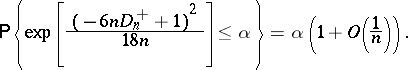The testing ofagainst the alternative:is dealt with similarly. In this case the statistic of the Kolmogorov–Smirnov test is the random variablewhose distribution is the same as that of the statisticwhenis true.

How to Cite This Entry:
Kolmogorov-Smirnov test. Encyclopedia of Mathematics. URL: http://encyclopediaofmath.org/index.php?title=Kolmogorov-Smirnov_test&oldid=17054
This article was adapted from an original article by M.S. Nikulin (originator), which appeared in Encyclopedia of Mathematics - ISBN 1402006098. See original article